# Mean State

Period Mean (original grids) [Watt m-2]
Model Period Mean (intersection) [Watt m-2]
Model Period Mean (complement) [Watt m-2]
Benchmark Period Mean (intersection) [Watt m-2]
Benchmark Period Mean (complement) [Watt m-2]
Bias [Watt m-2]
RMSE [Watt m-2]
Phase Shift [months]
Bias Score 
RMSE Score 
Seasonal Cycle Score 
Spatial Distribution Score 
Interannual Variability Score 
Overall Score 
Benchmark [-] 217.
CLM4 [-] 228. 227. 217. 232. 11.0 22.1 0.909 0.580 0.471 0.873 0.992 0.520 0.651
CLM4.5 [-] 228. 227. 217. 232. 11.0 22.1 0.909 0.580 0.471 0.873 0.992 0.520 0.651
CLM5 [-] 228. 227. 217. 232. 11.0 22.1 0.909 0.580 0.471 0.873 0.992 0.520 0.651
Period Mean (original grids) [Watt m-2]
Model Period Mean (intersection) [Watt m-2]
Model Period Mean (complement) [Watt m-2]
Benchmark Period Mean (intersection) [Watt m-2]
Benchmark Period Mean (complement) [Watt m-2]
Bias [Watt m-2]
RMSE [Watt m-2]
Phase Shift [months]
Bias Score 
RMSE Score 
Seasonal Cycle Score 
Spatial Distribution Score 
Interannual Variability Score 
Overall Score 
Benchmark [-] 241.
CLM4 [-] 245. 245. 241. 238. 4.28 22.8 0.581 0.648 0.524 0.951 0.929 0.663 0.706
CLM4.5 [-] 245. 245. 241. 238. 4.28 22.8 0.581 0.648 0.524 0.951 0.929 0.663 0.706
CLM5 [-] 245. 245. 241. 238. 4.28 22.8 0.581 0.648 0.524 0.951 0.929 0.663 0.706
Period Mean (original grids) [Watt m-2]
Model Period Mean (intersection) [Watt m-2]
Model Period Mean (complement) [Watt m-2]
Benchmark Period Mean (intersection) [Watt m-2]
Benchmark Period Mean (complement) [Watt m-2]
Bias [Watt m-2]
RMSE [Watt m-2]
Phase Shift [months]
Bias Score 
RMSE Score 
Seasonal Cycle Score 
Spatial Distribution Score 
Interannual Variability Score 
Overall Score 
Benchmark [-] 233.
CLM4 [-] 231. 231. 233. 238. -1.27 21.2 0.855 0.727 0.552 0.892 0.906 0.548 0.696
CLM4.5 [-] 231. 231. 233. 238. -1.27 21.2 0.855 0.727 0.552 0.892 0.906 0.548 0.696
CLM5 [-] 231. 231. 233. 238. -1.27 21.2 0.855 0.727 0.552 0.892 0.906 0.548 0.696
Period Mean (original grids) [Watt m-2]
Model Period Mean (intersection) [Watt m-2]
Model Period Mean (complement) [Watt m-2]
Benchmark Period Mean (intersection) [Watt m-2]
Benchmark Period Mean (complement) [Watt m-2]
Bias [Watt m-2]
RMSE [Watt m-2]
Phase Shift [months]
Bias Score 
RMSE Score 
Seasonal Cycle Score 
Spatial Distribution Score 
Interannual Variability Score 
Overall Score 
Benchmark [-] 111.
CLM4 [-] 112. 112. 111. 117. 0.518 16.3 0.0404 0.936 0.826 0.997 0.992 0.507 0.847
CLM4.5 [-] 112. 112. 111. 117. 0.518 16.3 0.0404 0.936 0.826 0.997 0.992 0.507 0.847
CLM5 [-] 112. 112. 111. 117. 0.518 16.3 0.0404 0.936 0.826 0.997 0.992 0.507 0.847
Period Mean (original grids) [Watt m-2]
Model Period Mean (intersection) [Watt m-2]
Model Period Mean (complement) [Watt m-2]
Benchmark Period Mean (intersection) [Watt m-2]
Benchmark Period Mean (complement) [Watt m-2]
Bias [Watt m-2]
RMSE [Watt m-2]
Phase Shift [months]
Bias Score 
RMSE Score 
Seasonal Cycle Score 
Spatial Distribution Score 
Interannual Variability Score 
Overall Score 
Benchmark [-] 213.
CLM4 [-] 216. 215. 209. 225. 9.33 24.3 1.51 0.558 0.429 0.778 0.905 0.494 0.599
CLM4.5 [-] 216. 215. 209. 225. 9.33 24.3 1.51 0.558 0.429 0.778 0.905 0.494 0.599
CLM5 [-] 216. 215. 209. 225. 9.33 24.3 1.51 0.558 0.429 0.778 0.905 0.494 0.599
Period Mean (original grids) [Watt m-2]
Model Period Mean (intersection) [Watt m-2]
Model Period Mean (complement) [Watt m-2]
Benchmark Period Mean (intersection) [Watt m-2]
Benchmark Period Mean (complement) [Watt m-2]
Bias [Watt m-2]
RMSE [Watt m-2]
Phase Shift [months]
Bias Score 
RMSE Score 
Seasonal Cycle Score 
Spatial Distribution Score 
Interannual Variability Score 
Overall Score 
Benchmark [-] 126.
CLM4 [-] 134. 134. 126. 145. 7.98 18.7 0.157 0.891 0.817 0.989 0.996 0.577 0.848
CLM4.5 [-] 134. 134. 126. 145. 7.98 18.7 0.157 0.891 0.817 0.989 0.996 0.577 0.848
CLM5 [-] 134. 134. 126. 145. 7.98 18.7 0.157 0.891 0.817 0.989 0.996 0.577 0.848
Period Mean (original grids) [Watt m-2]
Model Period Mean (intersection) [Watt m-2]
Model Period Mean (complement) [Watt m-2]
Benchmark Period Mean (intersection) [Watt m-2]
Benchmark Period Mean (complement) [Watt m-2]
Bias [Watt m-2]
RMSE [Watt m-2]
Phase Shift [months]
Bias Score 
RMSE Score 
Seasonal Cycle Score 
Spatial Distribution Score 
Interannual Variability Score 
Overall Score 
Benchmark [-] 170.
CLM4 [-] 188. 187. 170. 169. 16.8 27.8 0.502 0.723 0.736 0.959 0.893 0.618 0.777
CLM4.5 [-] 188. 187. 170. 169. 16.8 27.8 0.502 0.723 0.736 0.959 0.893 0.618 0.777
CLM5 [-] 188. 187. 170. 169. 16.8 27.8 0.502 0.723 0.736 0.959 0.893 0.618 0.777
Period Mean (original grids) [Watt m-2]
Model Period Mean (intersection) [Watt m-2]
Model Period Mean (complement) [Watt m-2]
Benchmark Period Mean (intersection) [Watt m-2]
Benchmark Period Mean (complement) [Watt m-2]
Bias [Watt m-2]
RMSE [Watt m-2]
Phase Shift [months]
Bias Score 
RMSE Score 
Seasonal Cycle Score 
Spatial Distribution Score 
Interannual Variability Score 
Overall Score 
Benchmark [-] 210.
CLM4 [-] 231. 231. 209. 224. 21.5 32.3 0.302 0.561 0.586 0.978 0.990 0.539 0.707
CLM4.5 [-] 231. 231. 209. 224. 21.5 32.3 0.302 0.561 0.586 0.978 0.990 0.539 0.707
CLM5 [-] 231. 231. 209. 224. 21.5 32.3 0.302 0.561 0.586 0.978 0.990 0.539 0.707
Period Mean (original grids) [Watt m-2]
Model Period Mean (intersection) [Watt m-2]
Model Period Mean (complement) [Watt m-2]
Benchmark Period Mean (intersection) [Watt m-2]
Benchmark Period Mean (complement) [Watt m-2]
Bias [Watt m-2]
RMSE [Watt m-2]
Phase Shift [months]
Bias Score 
RMSE Score 
Seasonal Cycle Score 
Spatial Distribution Score 
Interannual Variability Score 
Overall Score 
Benchmark [-] 105.
CLM4 [-] 103. 104. 105. 108. -1.87 18.0 0.284 0.918 0.796 0.981 0.901 0.517 0.818
CLM4.5 [-] 103. 104. 105. 108. -1.87 18.0 0.284 0.918 0.796 0.981 0.901 0.517 0.818
CLM5 [-] 103. 104. 105. 108. -1.87 18.0 0.284 0.918 0.796 0.981 0.901 0.517 0.818
Period Mean (original grids) [Watt m-2]
Model Period Mean (intersection) [Watt m-2]
Model Period Mean (complement) [Watt m-2]
Benchmark Period Mean (intersection) [Watt m-2]
Benchmark Period Mean (complement) [Watt m-2]
Bias [Watt m-2]
RMSE [Watt m-2]
Phase Shift [months]
Bias Score 
RMSE Score 
Seasonal Cycle Score 
Spatial Distribution Score 
Interannual Variability Score 
Overall Score 
Benchmark [-] 228.
CLM4 [-] 235. 235. 229. 216. 2.90 18.7 0.429 0.877 0.736 0.971 0.970 0.654 0.824
CLM4.5 [-] 235. 235. 229. 216. 2.90 18.7 0.429 0.877 0.736 0.971 0.970 0.654 0.824
CLM5 [-] 235. 235. 229. 216. 2.90 18.7 0.429 0.877 0.736 0.971 0.970 0.654 0.824
Period Mean (original grids) [Watt m-2]
Model Period Mean (intersection) [Watt m-2]
Model Period Mean (complement) [Watt m-2]
Benchmark Period Mean (intersection) [Watt m-2]
Benchmark Period Mean (complement) [Watt m-2]
Bias [Watt m-2]
RMSE [Watt m-2]
Phase Shift [months]
Bias Score 
RMSE Score 
Seasonal Cycle Score 
Spatial Distribution Score 
Interannual Variability Score 
Overall Score 
Benchmark [-] 140.
CLM4 [-] 158. 158. 138. 161. 20.2 28.5 0.194 0.752 0.799 0.987 0.997 0.642 0.829
CLM4.5 [-] 158. 158. 138. 161. 20.2 28.5 0.194 0.752 0.799 0.987 0.997 0.642 0.829
CLM5 [-] 158. 158. 138. 161. 20.2 28.5 0.194 0.752 0.799 0.987 0.997 0.642 0.829
Period Mean (original grids) [Watt m-2]
Model Period Mean (intersection) [Watt m-2]
Model Period Mean (complement) [Watt m-2]
Benchmark Period Mean (intersection) [Watt m-2]
Benchmark Period Mean (complement) [Watt m-2]
Bias [Watt m-2]
RMSE [Watt m-2]
Phase Shift [months]
Bias Score 
RMSE Score 
Seasonal Cycle Score 
Spatial Distribution Score 
Interannual Variability Score 
Overall Score 
Benchmark [-] 191.
CLM4 [-] 190. 190. 186. 193. 4.01 23.2 0.455 0.761 0.685 0.952 0.984 0.544 0.769
CLM4.5 [-] 190. 190. 186. 193. 4.01 23.2 0.455 0.761 0.685 0.952 0.984 0.544 0.769
CLM5 [-] 191. 191. 186. 193. 4.01 23.2 0.455 0.761 0.685 0.952 0.984 0.544 0.769
Period Mean (original grids) [Watt m-2]
Model Period Mean (intersection) [Watt m-2]
Model Period Mean (complement) [Watt m-2]
Benchmark Period Mean (intersection) [Watt m-2]
Benchmark Period Mean (complement) [Watt m-2]
Bias [Watt m-2]
RMSE [Watt m-2]
Phase Shift [months]
Bias Score 
RMSE Score 
Seasonal Cycle Score 
Spatial Distribution Score 
Interannual Variability Score 
Overall Score 
Benchmark [-] 240.
CLM4 [-] 249. 249. 240. 241. 8.59 22.4 0.367 0.809 0.736 0.970 0.991 0.613 0.809
CLM4.5 [-] 249. 249. 240. 241. 8.59 22.4 0.367 0.809 0.736 0.970 0.991 0.613 0.809
CLM5 [-] 249. 249. 240. 241. 8.59 22.4 0.367 0.809 0.736 0.970 0.991 0.613 0.809
Period Mean (original grids) [Watt m-2]
Model Period Mean (intersection) [Watt m-2]
Model Period Mean (complement) [Watt m-2]
Benchmark Period Mean (intersection) [Watt m-2]
Benchmark Period Mean (complement) [Watt m-2]
Bias [Watt m-2]
RMSE [Watt m-2]
Phase Shift [months]
Bias Score 
RMSE Score 
Seasonal Cycle Score 
Spatial Distribution Score 
Interannual Variability Score 
Overall Score 
Benchmark [-] 113.
CLM4 [-] 117. 117. 113. 116. 3.88 18.4 0.110 0.894 0.817 0.993 0.794 0.480 0.799
CLM4.5 [-] 117. 117. 113. 116. 3.88 18.4 0.110 0.894 0.817 0.993 0.794 0.480 0.799
CLM5 [-] 117. 117. 113. 116. 3.88 18.4 0.110 0.894 0.817 0.993 0.794 0.480 0.799
Period Mean (original grids) [Watt m-2]
Model Period Mean (intersection) [Watt m-2]
Model Period Mean (complement) [Watt m-2]
Benchmark Period Mean (intersection) [Watt m-2]
Benchmark Period Mean (complement) [Watt m-2]
Bias [Watt m-2]
RMSE [Watt m-2]
Phase Shift [months]
Bias Score 
RMSE Score 
Seasonal Cycle Score 
Spatial Distribution Score 
Interannual Variability Score 
Overall Score 
Benchmark [-] 229.
CLM4 [-] 223. 223. 227. 241. -2.57 26.4 0.757 0.668 0.630 0.924 0.704 0.567 0.687
CLM4.5 [-] 223. 223. 227. 241. -2.57 26.4 0.757 0.668 0.630 0.924 0.704 0.567 0.687
CLM5 [-] 223. 223. 227. 241. -2.57 26.4 0.757 0.668 0.630 0.924 0.704 0.567 0.687
Period Mean (original grids) [Watt m-2]
Model Period Mean (intersection) [Watt m-2]
Model Period Mean (complement) [Watt m-2]
Benchmark Period Mean (intersection) [Watt m-2]
Benchmark Period Mean (complement) [Watt m-2]
Bias [Watt m-2]
RMSE [Watt m-2]
Phase Shift [months]
Bias Score 
RMSE Score 
Seasonal Cycle Score 
Spatial Distribution Score 
Interannual Variability Score 
Overall Score 
Benchmark [-] 173.
CLM4 [-] 186. 186. 172. 207. 15.1 22.2 0.367 0.775 0.784 0.976 0.984 0.503 0.801
CLM4.5 [-] 186. 186. 172. 207. 15.1 22.2 0.367 0.775 0.784 0.976 0.984 0.503 0.801
CLM5 [-] 186. 186. 172. 207. 15.1 22.2 0.367 0.775 0.784 0.976 0.984 0.503 0.801
Period Mean (original grids) [Watt m-2]
Model Period Mean (intersection) [Watt m-2]
Model Period Mean (complement) [Watt m-2]
Benchmark Period Mean (intersection) [Watt m-2]
Benchmark Period Mean (complement) [Watt m-2]
Bias [Watt m-2]
RMSE [Watt m-2]
Phase Shift [months]
Bias Score 
RMSE Score 
Seasonal Cycle Score 
Spatial Distribution Score 
Interannual Variability Score 
Overall Score 
Benchmark [-] 135.
CLM4 [-] 149. 149. 135. 133. 13.8 20.4 0.186 0.830 0.825 0.988 0.959 0.544 0.829
CLM4.5 [-] 149. 149. 135. 133. 13.8 20.4 0.186 0.830 0.825 0.988 0.959 0.544 0.829
CLM5 [-] 149. 149. 135. 133. 13.8 20.4 0.186 0.830 0.825 0.988 0.959 0.544 0.829
Period Mean (original grids) [Watt m-2]
Model Period Mean (intersection) [Watt m-2]
Model Period Mean (complement) [Watt m-2]
Benchmark Period Mean (intersection) [Watt m-2]
Benchmark Period Mean (complement) [Watt m-2]
Bias [Watt m-2]
RMSE [Watt m-2]
Phase Shift [months]
Bias Score 
RMSE Score 
Seasonal Cycle Score 
Spatial Distribution Score 
Interannual Variability Score 
Overall Score 
Benchmark [-] 193.
CLM4 [-] 191. 191. 193. 181. -1.86 16.9 0.233 0.923 0.814 0.984 0.975 0.545 0.843
CLM4.5 [-] 191. 191. 193. 181. -1.86 16.9 0.233 0.923 0.814 0.984 0.975 0.545 0.843
CLM5 [-] 191. 191. 193. 181. -1.86 16.9 0.233 0.923 0.814 0.984 0.975 0.545 0.843
Period Mean (original grids) [Watt m-2]
Model Period Mean (intersection) [Watt m-2]
Model Period Mean (complement) [Watt m-2]
Benchmark Period Mean (intersection) [Watt m-2]
Benchmark Period Mean (complement) [Watt m-2]
Bias [Watt m-2]
RMSE [Watt m-2]
Phase Shift [months]
Bias Score 
RMSE Score 
Seasonal Cycle Score 
Spatial Distribution Score 
Interannual Variability Score 
Overall Score 
Benchmark [-] 202.
CLM4 [-] 211. 211. 201. 241. 10.1 21.6 1.24 0.608 0.461 0.829 0.909 0.514 0.630
CLM4.5 [-] 211. 211. 201. 241. 10.1 21.6 1.24 0.608 0.461 0.829 0.909 0.514 0.630
CLM5 [-] 211. 211. 201. 241. 10.1 21.6 1.24 0.608 0.461 0.829 0.909 0.514 0.630
Period Mean (original grids) [Watt m-2]
Model Period Mean (intersection) [Watt m-2]
Model Period Mean (complement) [Watt m-2]
Benchmark Period Mean (intersection) [Watt m-2]
Benchmark Period Mean (complement) [Watt m-2]
Bias [Watt m-2]
RMSE [Watt m-2]
Phase Shift [months]
Bias Score 
RMSE Score 
Seasonal Cycle Score 
Spatial Distribution Score 
Interannual Variability Score 
Overall Score 
Benchmark [-] 211.
CLM4 [-] 217. 217. 211. 216. 5.51 23.6 0.836 0.770 0.584 0.903 0.840 0.488 0.695
CLM4.5 [-] 217. 217. 211. 216. 5.51 23.6 0.836 0.770 0.584 0.903 0.840 0.488 0.695
CLM5 [-] 217. 217. 211. 216. 5.51 23.6 0.836 0.770 0.584 0.903 0.840 0.488 0.695
Period Mean (original grids) [Watt m-2]
Model Period Mean (intersection) [Watt m-2]
Model Period Mean (complement) [Watt m-2]
Benchmark Period Mean (intersection) [Watt m-2]
Benchmark Period Mean (complement) [Watt m-2]
Bias [Watt m-2]
RMSE [Watt m-2]
Phase Shift [months]
Bias Score 
RMSE Score 
Seasonal Cycle Score 
Spatial Distribution Score 
Interannual Variability Score 
Overall Score 
Benchmark [-] 204.
CLM4 [-] 210. 209. 205. 190. 1.38 32.0 0.437 0.698 0.686 0.956 0.764 0.522 0.719
CLM4.5 [-] 210. 209. 205. 190. 1.38 32.0 0.437 0.698 0.686 0.956 0.764 0.522 0.719
CLM5 [-] 210. 209. 205. 190. 1.38 32.0 0.437 0.698 0.686 0.956 0.764 0.522 0.719

# Temporally integrated period mean

BENCHMARK MEAN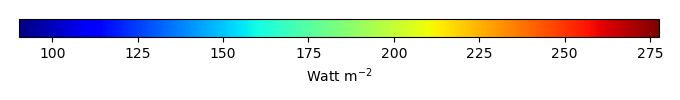MODEL MEANBIAS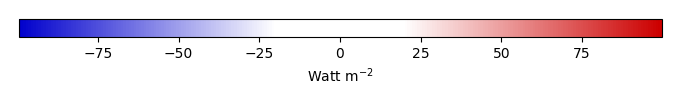BIAS SCORERMSE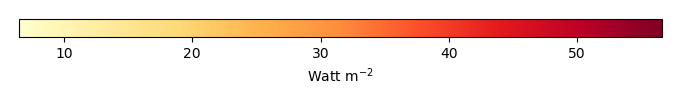RMSE SCOREBENCHMARK INTERANNUAL VARIABILITY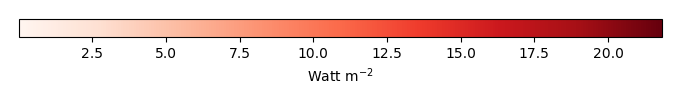MODEL INTERANNUAL VARIABILITYINTERANNUAL VARIABILITY SCOREBENCHMARK MAX MONTHMODEL MAX MONTHDIFFERENCE IN MAX MONTH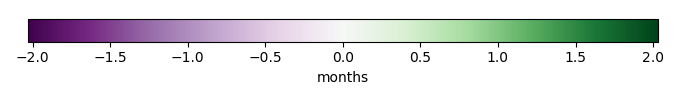SEASONAL CYCLE SCORESPATIAL TAYLOR DIAGRAMMODEL COLORS# Spatially integrated regional mean

MODEL COLORSREGIONAL MEANANNUAL CYCLEMONTHLY ANOMALYANNUAL CYCLE# All Models

BenchmarkCLM4CLM4.5CLM5# Data Information

creation_date: Mon Jun 30 23:16:59 PDT 2014

source_file: This product is generated from monthly 1 degree GEWEX SRB Radiation observations